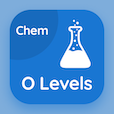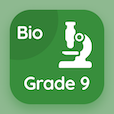Cambridge Online Courses (GCSE)

O Level Chemistry Quizzes

O Level Chemistry Quiz PDF - Complete

# Proton and Nucleon Number Multiple Choice Questions p. 104

Study Proton and Nucleon Number multiple choice questions and answers, proton and nucleon number quiz answers PDF 104 to study O Level Chemistry course online. Structure of Atom MCQ trivia questions, proton and nucleon number Multiple Choice Questions (MCQ) for online college degrees. "Proton and Nucleon Number MCQ" PDF eBook: chemical and ionic equations, salts: hydrogen of acids, proton and nucleon number test prep for online college courses.

"In Hydrogen (H), the number of neutrons is" MCQ PDF: 1, 2, 0, and depends upon the type of bond it enters for best GRE prep courses online. Learn structure of atom questions and answers to improve problem solving skills to learn free online courses.

## Proton & Nucleon Number Questions and Answers MCQs

MCQ: In Hydrogen (H), the number of neutrons is

2
1
0
depends upon the type of bond it enters

MCQ: Consider the reaction: AgNO3 + NaCl → AgCl + NaNO3. The state of AgCl will be

solid
aqueous
gas
liquid

MCQ: Chemical formula of Copper(I) Oxide

Cu2O
CuO3
Cu(OH)2
CuO

MCQ: If waters relative molecular mass is 36, the molecular formula will turn out to be

H2O
H2O2
H2O4
H8O4

MCQ: If the number of electrons in chlorine is 17, number of shells required will be

2
3
4
5

### More Quizzes from O Level Chemistry Course